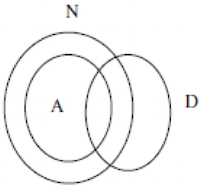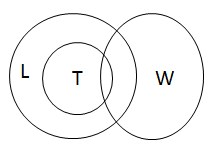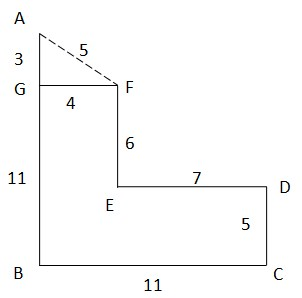# HCL Technologies Reasoning Questions

DIRECTIONS for the question 1 to 2: The question below has either two or three statements followed by two or three conclusions. You have to take the given statements to be true and then decide which of the given conclusions logically follows from the given statements, disregarding the commonly known facts.
1. Statements: All alphabets are numbers, some alphabets are digits
Conclusions: I. At least some digits are numbers
II. No digit is a number
1. Either conclusion I or II follows
2. Neither conclusion I nor II follows
3. Only conclusion II follows
4. Only conclusion I follow
5. Both conclusions I and II follow
From the given statements, we get the following relation: Therefore,Conclusion I: At least some digits are numbers is true
Conclusion II: No digit is a number is not true
So the correct option is D.
2. Statements: Some wins are losses, All trophies are losses.
Conclusions: I. All trophies are wins
II. All losses are trophies
1. Either conclusion I or II follows
2. Both conclusions I and II follow
3. Only conclusion II follows
4. Only conclusion I follow
5. Neither conclusion I nor II follows
From the given statements, we get the following relation: Therefore,Conclusion I: All trophies are wins is not true.
Conclusion II: All losses are trophies is also not true. Some losses are trophies
So the correct option is E.
DIRECTIONS for the question 3 to 5: Read the information given below and answer the question that follows.
A, B, C, D, E, F and G are standing in a straight line facing north with equal distance between them, not necessary in the same order. Each one is pursuing a different profession – actor, reporter, doctor, engineer, lawyer, teacher and painter not necessary in the same order.
G is fifth to the left of C. The reporter is third to the right of G. F is fifth to the right of A. E is second to the left of B. The engineer is second to the left of D. There are only three people between the engineer and the painter. The doctor is to the immediate left of the engineer. The lawyer is to the immediate right of the teacher.
1. Who amongst the following is the actor?
1. E
2. F
3. C
4. B
5. A
From the given information, we have the following arrangement :
 A G E D B F C DOCTOR ENGINEER TEACHER LAWYER REPORTER PAINTER ACTOR

C is the actor. Hence option C.
2. What is D’s position with respect to the painter?
1. Third to the left
2. Second to the right
3. Fourth to the right
4. Third to the right
5. Second to the left
From the given information, we have the following arrangement :
 A G E D B F C DOCTOR ENGINEER TEACHER LAWYER REPORTER PAINTER ACTOR

D’s position with respect to the painter is second to the left. Hence option E.
3. Who among the following are standing at the extremes?
1. A and F
2. G and C
3. G and F
4. A and B
5. A and C
From the given information, we have the following arrangement :
 A G E D B F C DOCTOR ENGINEER TEACHER LAWYER REPORTER PAINTER ACTOR

A and C are standing at the extremes. Hence option E.
DIRECTIONS for the question 6: Solve the following question and mark the best possible option.
In a certain code,
‘very large risk associated’ is written as ‘nu ta ro gi’
‘risk is very low’ is written as ‘ gi se nu mi’
‘is that also associated’ is written as ‘ta mi po fu’
‘inherent risk also damaging’ is written as ‘fu nu di yu’
(All the codes are two letter codes only.)
4. What does the code ‘di’ stand for?
1. Either ‘damaging’ or ‘inherent’
2. Inherent
3. Also
4. low
5. risk
‘very large risk associated’ is written as ‘nu ta ro gi’-(1)
‘risk is very low’ is written as ‘ gi se nu mi-----(2)
‘is that also associated’ is written as ‘ta mi po fu’----(3)
‘inherent risk also damaging’ is written as ‘fu nu di yu’---(4)
From statements 1 and 3, we have ‘associated’ as the common word and the common code is ‘ta’
From statements 3 and 4, we have ‘also’ as the common word and the common code is ‘fu’
From statements 2 and 4, we have ‘risk’ as the common word and the common code is ‘nu’
From statements 2 and 3, we have ‘is’ as the common word and the common code is ‘mi’
From statements 1 and 2, we can conclude that the code of ‘very’ is ‘gi’
Therefore, from statement 1, the code of ‘large’ will be ‘ro’
Similarly, from statement 2, the code of ‘low’ is ‘se’ and from statement 3, the code of ‘that’ is ‘po’
 Word Code very gi risk nu is mi also fu low se large ro that po associated ta inherient di/yu damaging di/yu

‘di’ can either be ‘damaging’ or ‘inherent’. Hence option A.
DIRECTIONS for the question 7: Solve the following question and mark the best possible option.
Point A is 14m north of point B
Point C is 11m east of point B
Point D is 5m north of point C
Point E is 7m west of point D
Point F is 6m north of point E
Point G is 4m west of point F
5. How far is F from point A ?
1. 5m
2. 5.67m
3. 6m
4. 3m
5. 4m
The distance between F and A i.eFA = √(AG2 + GF2) = √(32 + 42) = √25 = 5m.
DIRECTIONS for the questions 8: The question below consist of a question and two statements numbered I and II given below it. You have to decide whether the data provided in the statements are sufficient to answer the question. Read both the statements and mark.
6. How many students are there in the class?
I. There are more than 20 but less than 27 students in the class.
II. There are more than 24 but less than 31 students in the class. the number of students in the class can be divided into groups such that each group contains 5 students.
1. The data in both the Statements I and II together are necessary to answer the question.
2. The data neither in Statement I nor in Statement II are sufficient to answer the question
3. The data either in Statement I alone or in Statement II alone are sufficient to answer the question
4. The data in Statement I alone are sufficient to answer the question, while the data in Statement II alone are not sufficient to answer the question
5. The data in Statement II alone are sufficient to answer the question, while the data in Statement I alone are not sufficient to answer the question.
From statement I, the no of students can be 21,22,23,24,25 or 26. So no certain answer is obtained.
From statement II, the no of students can be either 25 or 30. So again no unique answer is obtained.
On combining the statements, we get 25 as the unique answer.
Therefore, the data in both the Statements I and II together are necessary to answer the question. Hence option A.
DIRECTIONS for the questions 9-10: Solve the following question and mark the best possible option.
7. Statement: K > I > T > E; O < R < K
Conclusion: I. R < E
II. O < T
1. Neither conclusion I nor II follows
2. Both conclusions I and II follows
3. Only conclusion II follows
4. Either conclusion I or II follows
5. Only conclusion I follows
Statement: K > I > T > E; O < R < K
Conclusion I: R < E is false because no relationship can be established between R & E.
Conclusion II: O < T is also false because no relationship can be established between O & T.
Thus, neither conclusion I nor II follows. Hence option A.
8. Statement: C < L < O = U = D > S > Y
Conclusion: I. O > Y
II. C < D
1. Neither conclusion I nor II follows
2. Both conclusions I and II follows
3. Only conclusion I follows
4. Only conclusion II follows
5. Either conclusion I or II follows